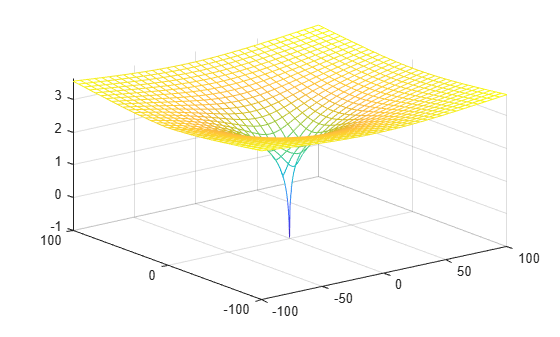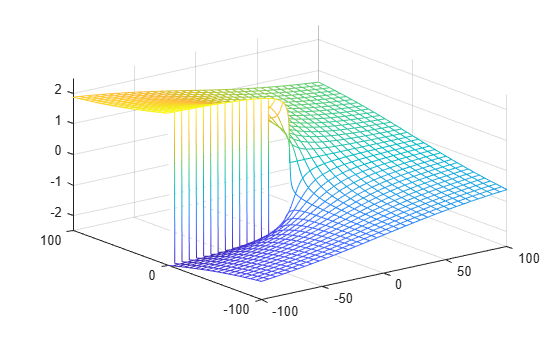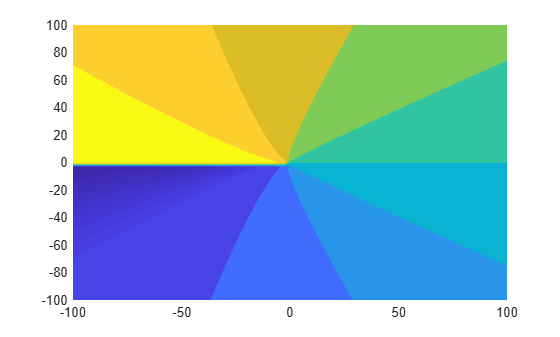# lambertw

Lambert W function

## Syntax

``lambertw(x)``
``lambertw(k,x)``

## Description

example

````lambertw(x)` returns the principal branch of the Lambert W function. This syntax is equivalent to `lambertw(0,x)`.```

example

````lambertw(k,x)` is the `k`th branch of the Lambert W function. This syntax returns real values only if `k = 0` or `k = -1`.```

## Examples

collapse all

The Lambert W function `W(x)` is a set of solutions of the equation `x = W(x)eW(x)`.

Solve this equation. The solution is the Lambert W function.

```syms x W eqn = x == W*exp(W); solve(eqn,W)```
```ans = lambertw(0, x)```

Verify that branches of the Lambert W function are valid solutions of the equation `x = W*eW`:

```k = -2:2; eqn = subs(eqn,W,lambertw(k,x)); isAlways(eqn)```
```ans = 1×5 logical array 1 1 1 1 1```

Depending on its arguments, `lambertw` can return floating-point or exact symbolic results.

Compute the Lambert W functions for these numbers. Because the numbers are not symbolic objects, you get floating-point results.

```A = [0 -1/exp(1); pi i]; lambertw(A)```
```ans = 0.0000 + 0.0000i -1.0000 + 0.0000i 1.0737 + 0.0000i 0.3747 + 0.5764i ```
`lambertw(-1,A)`
```ans = -Inf + 0.0000i -1.0000 + 0.0000i -0.3910 - 4.6281i -1.0896 - 2.7664i```

Compute the Lambert W functions for the numbers converted to symbolic objects. For most symbolic (exact) numbers, `lambertw` returns unresolved symbolic calls.

```A = [0 -1/exp(sym(1)); pi i]; W0 = lambertw(A)```
```W0 = [ 0, -1] [ lambertw(0, pi), lambertw(0, 1i)] ```
`Wmin1 = lambertw(-1,A)`
```Wmin1 = [ -Inf, -1] [ lambertw(-1, pi), lambertw(-1, 1i)]```

Convert symbolic results to double by using `double`.

`double(W0)`
```ans = 0.0000 + 0.0000i -1.0000 + 0.0000i 1.0737 + 0.0000i 0.3747 + 0.5764i```

Plot the two main branches, ${W}_{0}\left(x\right)$ and ${W}_{-1}\left(x\right)$, of the Lambert W function.

```syms x fplot(lambertw(x)) hold on fplot(lambertw(-1,x)) hold off axis([-0.5 4 -4 2]) title('Lambert W function, two main branches') legend('k=0','k=1','Location','best')```Plot the principal branch of the Lambert W function on the complex plane.

Plot the real value of the Lambert W function by using `fmesh`. Simultaneously plot the contours by setting `'ShowContours'` to `'On'`.

```syms x y f = lambertw(x + 1i*y); interval = [-100 100 -100 100]; fmesh(real(f),interval,'ShowContours','On')```Plot the imaginary value of the Lambert W function. The plot has a branch cut along the negative real axis. Plot the contours separately.

`fmesh(imag(f),interval)``fcontour(imag(f),interval,'Fill','on')`Plot the absolute value of the Lambert W function.

`fmesh(abs(f),interval,'ShowContours','On')`## Input Arguments

collapse all

Input, specified as a number, vector, matrix, or array, or a symbolic number, variable, array, function, or expression.

At least one input argument must be a scalar, or both arguments must be vectors or matrices of the same size. If one input argument is a scalar and the other is a vector or matrix, `lambertw` expands the scalar into a vector or matrix of the same size as the other argument with all elements equal to that scalar.

Branch of Lambert W function, specified as an integer, a vector or matrix of integers, a symbolic integer, or a symbolic vector or matrix of integers.

At least one input argument must be a scalar, or both arguments must be vectors or matrices of the same size. If one input argument is a scalar and the other is a vector or matrix, `lambertw` expands the scalar into a vector or matrix of the same size as the other argument with all elements equal to that scalar.

collapse all

### Lambert W Function

The Lambert W function W(x) represents the solutions y of the equation $y{e}^{y}=x$ for any complex number `x`.

• For complex x, the equation has an infinite number of solutions y = lambertW(k,x) where k ranges over all integers.

• For all real x ≥ 0, the equation has exactly one real solution y = lambertW(x) = lambertW(0,x).

• For real x where $-{e}^{-1}, the equation has exactly two real solutions. The larger solution is represented by y = lambertW(x) and the smaller solution by y = lambertW(–1,x).

• For $x=-{e}^{-1}$, the equation has exactly one real solution y = –1 = lambertW(0, –exp(–1)) = lambertW(–1, -exp(–1)).

 Corless, R.M., G.H. Gonnet, D.E.G. Hare, D.J. Jeffrey, and D.E. Knuth. "On the Lambert W Function." Advances in Computational Mathematics, Vol. 5, pp. 329–359, 1996.

## Support

#### Mathematical Modeling with Symbolic Math Toolbox

Get examples and videos